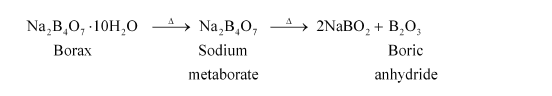# What happens whenQuestion:

What happens when

(a) Borax is heated strongly,

(b) Boric acid is added to water,

(c) Aluminium is treated with dilute NaOH,

(d) BF3 is reacted with ammonia?

Solution:

(a) When heated, borax undergoes various transitions. It first loses water molecules and swells. Then, it turns into a transparent liquid, solidifying to form a glass-like material called borax bead.(b) When boric acid is added to water, it accepts electrons from OH ion.

$\mathrm{B}(\mathrm{OH})_{3}+2 \mathrm{HOH} \longrightarrow\left[\mathrm{B}(\mathrm{OH})_{4}\right]^{-}+\mathrm{H}_{3} \mathrm{O}^{+}$

(c) Al reacts with dilute NaOH to form sodium tetrahydroxoaluminate(III). Hydrogen gas is liberated in the process.

$2 \mathrm{Al}_{(s)}+2 \mathrm{NaOH}_{(a q)}+6 \mathrm{H}_{2} \mathrm{O}_{(\ell)} \longrightarrow 2 \mathrm{Na}^{+}\left[\mathrm{Al}(\mathrm{OH})_{4}\right]_{(a q)}^{-}+3 \mathrm{H}_{2(g)}$

(d) BF3 (a Lewis acid) reacts with NH3 (a Lewis base) to form an adduct. This results in a complete octet around B in BF3.

$\mathrm{F}_{3} \mathrm{~B}+: \mathrm{NH}_{3} \longrightarrow \mathrm{F}_{3} \mathrm{~B} \leftarrow: \mathrm{NH}_{3}$